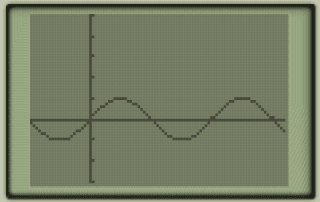# Graphing trigonometric functions

Trigonometric functions begin with a review of the unit circle and trig ratios. Y=sin(x) begins at the origin, peaks y=1, crosses the x-axis at pi, bottoms out at y=-1 and ends its first period at 2pi,0.y=sin(x) with x axis increments set to pi and y axis set to 1

Y=cos(x) starts at y=1, descends to cross the x-axis at pi/2, bottoms out at y=-1, crosses the x-axis at 3pi/2, and finishes its period at 2pi.y=cos(x) with x axis increments set to pi and y axis set to 1

Now, for all functions F(t)=A(Bt-C) + D where f(t) is a trig function, we know that: A: amplitude, B:period=2pi/|B|, C: phase shift is C/B and D: vertical shift